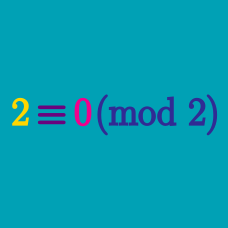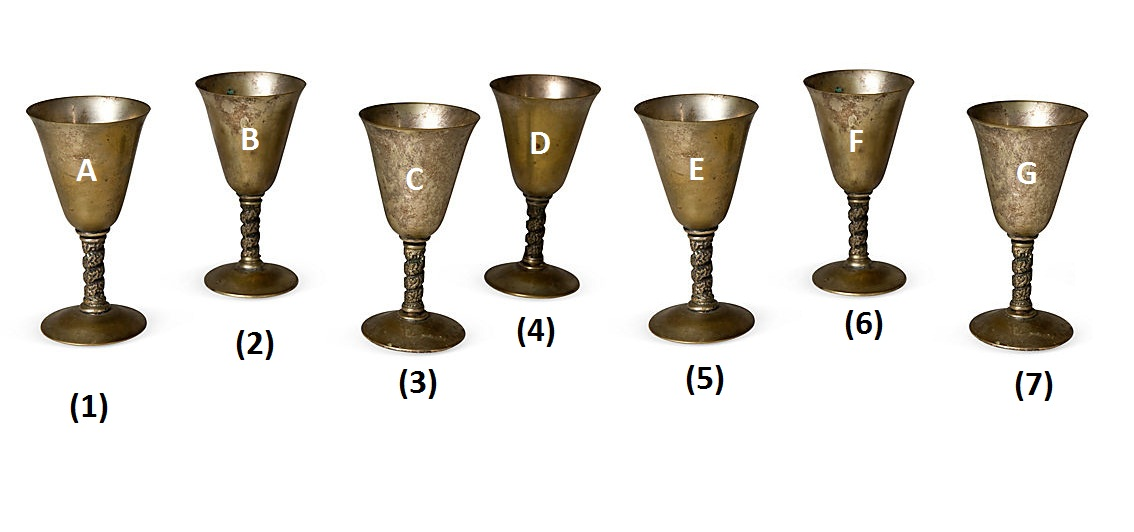Number Theory

# Modular Arithmetic Operations: Level 1 Challenges

What is the last digit when

$1234 \times 5678$

is multiplied out?

If today is a Monday, then what day will it be 100 days later?

$\LARGE \color{#D61F06} 5^{\color{#3D99F6}8^{\color{#20A900}{12}^{\color{#69047E}{15}^{\color{#624F41}{104}}}}} + \color{#D61F06}1$

Determine the smallest prime divisor of the gigantic number above.One of the seven goblets above is made of real gold. If you start counting at A and wind back and forth while counting (A, B, C, D, E, F, G, F, E, D, ...), then the golden goblet would be the $1000^\text{th}$ one that you count.

Which one is the golden goblet?

If $a$ is a negative odd number and $b$ is a positive even number, then which of the following must be a positive even number?

×

Problem Loading...

Note Loading...

Set Loading...